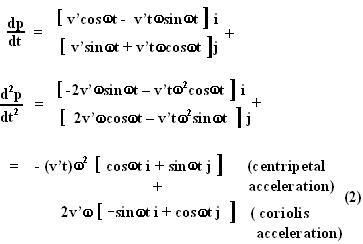## Friday, December 14, 2007

### Irodov Problem 1.112In this problem because the particle is moving linearly on a uniformly rotating disc it will experience the Coriolis psuedo force in the frame fixed to the particle's motion in addition to the centrifugal force. Centrifugal force is responsible for rotating the body around its center of rotation. It does not alter its magnitude. However, as the body moves radially outwards (or inwards) the magnitude of its tangential velocity must also change as it is given by angular speed times the radius. The Coriolis force is responsible to bring about this change in magnitude of tangential velocity.

Rather than using the expression for the force I will derive it from first principles so that the origin of the force is clear.

The position vector p of the particle (with the center of the disc as the origin) with respect to a stationary observer can be written as,Here, the particle is assumed to have started at the center and move radially outwards so it will be at a radius v't from the center. The nature or the value of the force will not change even if it started somewhere else rather than the center since it will simply be (v't - k) instead of v't and the constant will disappear when we differentiate.From the point of view of the observer on the moving particle both these accelerations will seem like forces acting in the opposite direction to the accelerations in (2).

The force exerted by the body on the particle on the disc is same as the reaction the disc exerts on the particle and this must provide for both the net acceleration of the particle. Further there is also the force of gravity that is acting on the body acting downwards with which the particle pushes the disc downwards. All three forces act in mutually perpendicular directions. Hence the net force exerted by the particle on the disc is given by,So while the centrifugal force is responsible for changing the direction of motion of the particle, the Coriolis force is responsible for changing the tangential velocity of the particle as it radius of rotation changes.